## Calculating the Effective Force (a more general derivation)

There are a lot of nasty derivations of the effective force in mechanics text books. We'd like to make ours simple yet not require too much higher mathematics, so we need to set up the problem as simply as possible.

Newton's laws apply in the inertial frame of reference. Lets work in that frame, but set up a set of unit vectors for an Accelerating frame. For simplicity of notation, lets call those unit vectors. Lets call the coordinates in the accelerating (rotating) frameand the position vector in the Inertial frameetc. Let the displacement between the origins of the Inertial and Accelerating frame be called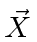. We then simply have.We will differentiate this equation twice to get accelerations and hence real and fictitious forces. Note that all the quantities in this equation are defined in the Inertial frame.The change ofwith time is a relative motion of the origins of the two coordinate systems. The change of the unit vectors with time is due to the rotation of the accelerating coordinate system.These are all the derivatives we need.

We also need the effect of the rotation on the unit vectors. In an infinitesimal timethe unit vectors rotate through an angle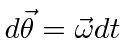.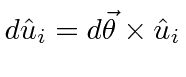This is the change of the unit vectors, evaluated in the inertial frame.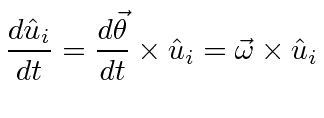We also need the second derivative.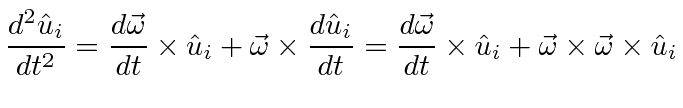We can now plug these into the formula for the second derivative ofabove.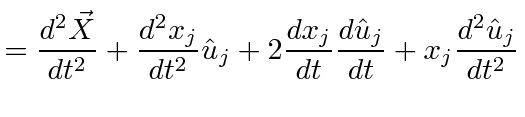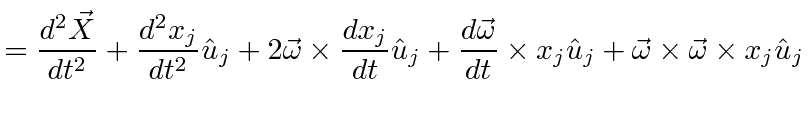We recognize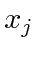as the position in the Accelerating frame (andas that position written in the inertial frame),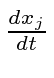as the velocity in the Accelerating frame, andas the acceleration seen in the Accelerating frame. It is important to note that these are written as vectors in the Inertial system by dotting them into the, which is why we can do this calculation rather easily. For example, thegive a position in the Accelerating frame, but when they are dotted into the basis vectors of the Accelerating frame, written in the Inertial frame,, the vector is now correctly written in the Inertial frame.In the equation above,is the acceleration the mass in the Inertial frame,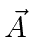is the acceleration of the origin of the Accelerating frame,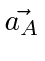is the acceleration of the mass seen in the Accelerating frame,is the velocity of the mass seen in the Accelerating frame,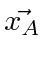is the position of the mass seen in the Accelerating frame, and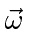is the angular velocity of the rotation of the coordinate axes of the accelerating (rotating) frame.andare properties of the transformation between the Inertial frame and the Accelerating frame.

This equation is written in the inertial frame but at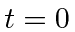the two frames coincide so the equation holds in the accelerating frame too. We can change to the accelerating frame just by removing the.

We can multiply by the massto get an equation in the forces, real and fictitious.Now solve this equation for the force seen in the Accelerating frame. The general equation for the effective force seen in a frame that is accelerating and rotating is.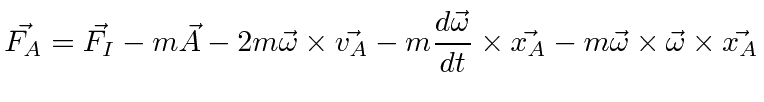There is a simple fictitious force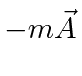if the coordinate system is simply accelerating. To see the effect of a constant rotation, we setandThe forces are named in the equation below.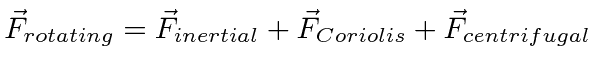The centrifugal and Coriolis forces are fictitious forces that appear in the rotating frame.

The Centrifugal Force grows with the perpendicular distance from the axis of rotation and is in the direction of.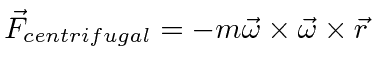The Coriolis Force is perpendicular to the velocity of the mass and to. Forpointing up, like in the northern hemisphere, the Coriolis force causes projectiles to deflect to the right. In the southern hemisphere, projectiles deflect to the left.Its interesting to note that Coriolis's original publication in 1835 was on the energy yield of machines with rotating parts, such as water-wheels.

Jim Branson 2012-10-21